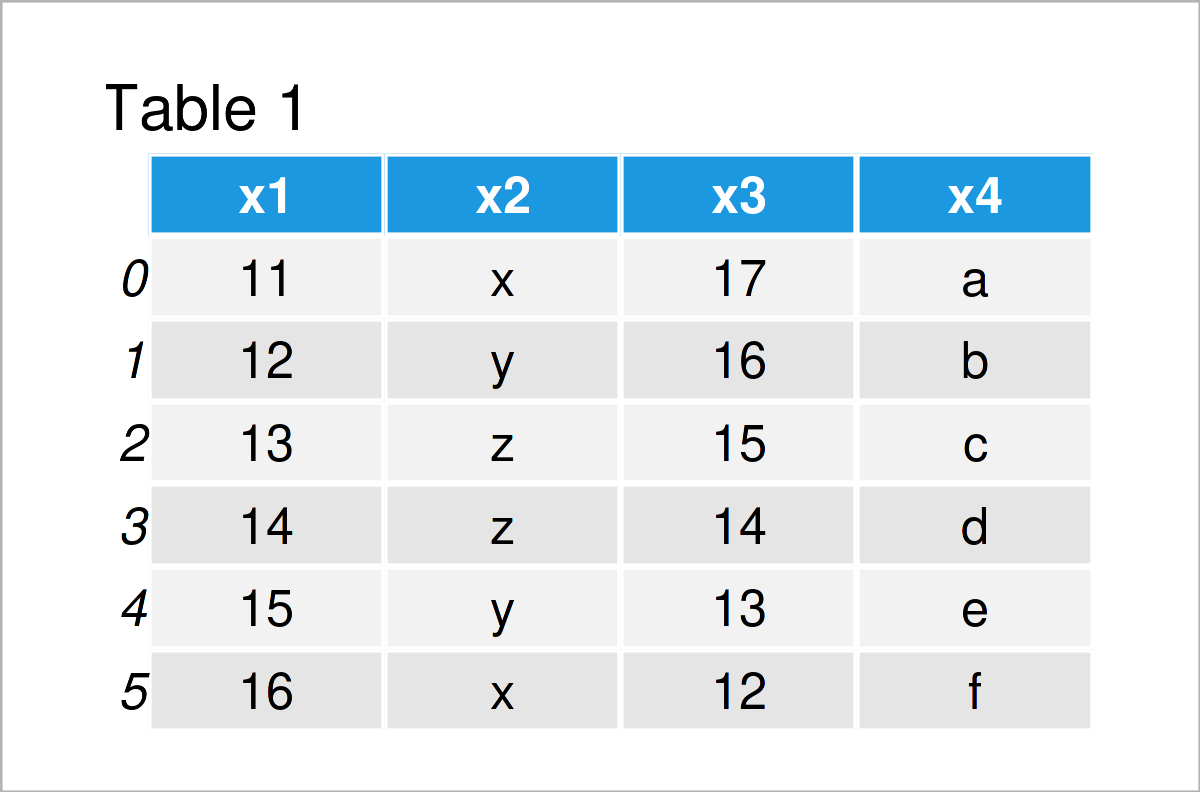# Specify dtype when Reading pandas DataFrame from CSV File in Python (Example)

In this tutorial you’ll learn how to set the data type for columns in a CSV file in Python programming.

The content of the post looks as follows:

So now the part you have been waiting for – the example:

## Example Data & Software Libraries

We first need to import the pandas library, to be able to use the corresponding functions:

`import pandas as pd                         # Import pandas library`

We use the following data as a basis for this Python programming tutorial:

```data = pd.DataFrame({'x1':range(11, 17),    # Create pandas DataFrame
'x2':['x', 'y', 'z', 'z', 'y', 'x'],
'x3':range(17, 11, - 1),
'x4':['a', 'b', 'c', 'd', 'e', 'f']})
print(data)                                 # Print pandas DataFrame```Table 1 shows the structure of our example data – It comprises six rows and four columns.

`data.to_csv('data.csv', index = False)      # Export pandas DataFrame to CSV`

After executing the previous code, a new CSV file should appear in your current working directory. We’ll use this file as a basis for the following example.

## Example: Set Data Type of Columns when Reading pandas DataFrame from CSV File

This example explains how to specify the data class of the columns of a pandas DataFrame when reading a CSV file into Python.

To accomplish this, we have to use the dtype argument within the read_csv function as shown in the following Python code. As you can see, we are specifying the column classes for each of the columns in our data set:

```data_import = pd.read_csv('data.csv',       # Import CSV file
dtype = {'x1': int, 'x2': str, 'x3': int, 'x4': str})```

The previous Python syntax has imported our CSV file with manually specified column classes.

Let’s check the classes of all the columns in our new pandas DataFrame:

```print(data_import.dtypes)                   # Check column classes of imported data
# x1     int32
# x2    object
# x3     int32
# x4    object
# dtype: object```

As you can see, the variables x1 and x3 are integers and the variables x2 and x4 are considered as string objects.

## Video, Further Resources & Summary

Would you like to learn more about the specification of the data type for variables in a CSV file? Then you could have a look at the following video on my YouTube channel. In the video, I’m explaining the examples of this tutorial.

Please accept YouTube cookies to play this video. By accepting you will be accessing content from YouTube, a service provided by an external third party.If you accept this notice, your choice will be saved and the page will refresh.

In addition, you may want to have a look at the related Python tutorials on this website. I have published numerous tutorials already:

To summarize: In this Python tutorial you have learned how to specify the data type for columns in a CSV file. Please let me know in the comments section below, in case you have any additional questions and/or comments on the pandas library or any other statistical topic.

Subscribe to the Statistics Globe Newsletter

•Keith
July 17, 2023 3:24 pm

Thanks for posting this. How do I specify the data type for only 1 column in a df using column number, not field name?

•July 18, 2023 7:24 am

Hello Keith,

If you’re reading the DataFrame from a CSV file, and you want to specify the datatype for only one column using its index, you have to do it in two steps because pandas’ read_csv function allows type specification only by column names, not by column indices. See the following please:

```import pandas as pd

#   brand     id   mois   prot    fat   ash  sodium  carb   cal
# 0     A  14069  27.82  21.43  44.87  5.11    1.77  0.77  4.93
# 1     A  14053  28.49  21.26  43.89  5.34    1.79  1.02  4.84
# 2     A  14025  28.35  19.99  45.78  5.08    1.63  0.80  4.95
# 3     A  14016  30.55  20.15  43.13  4.79    1.61  1.38  4.74
# 4     A  14005  30.49  21.28  41.65  4.82    1.64  1.76  4.67

# Get the column name using column index, e.g., for the first column (index 0)
column_name = df.columns

# Change the data type of the selected column, e.g., to 'str'
df[column_name] = df[column_name].astype('int')